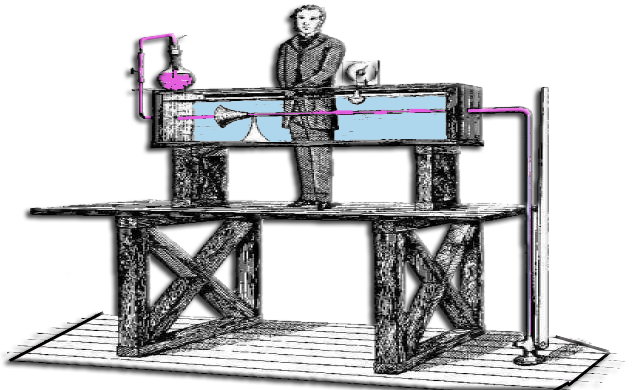• Reynolds gave us a bridge between the different fluids and different scales
Reynolds number is a dimensionless number linking viscosity, density, and a reference length. The reference length can be :

Re=V.L/u

Re = ((average speed) x (reference length)) / (kinematic viscosity of the fluid)

or also

Re = ((mass per units volume) x (average speed) x (reference length)) / (dynamic viscosity of the fluid)The Reynolds number is used to determine the flow regime, Laminar, Transitional and Turbulent and equations to use.